# Resistance Measurement 1 Resistance Measurement The resistances are

• Slides: 20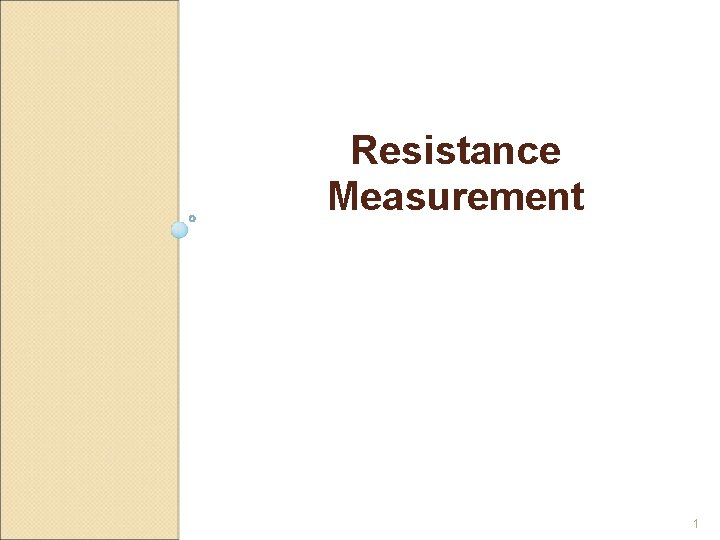Resistance Measurement 1Resistance Measurement � The resistances are classified as ; Ø 1. Low Resistance : All resistances of the order of 1 ohm and below. example: Machine armature, series field winding shunt etc. Ø 2. Medium Resistance : All resistances of the order of 1 ohm to 100, 000 ohms. example: Winding resistance, multiplier resistance. Ø 3. High Resistance : All resistances of the order of 100, 000 ohm and above. example: Insulation resistance of machines, cables, porcelain insulator etc. 2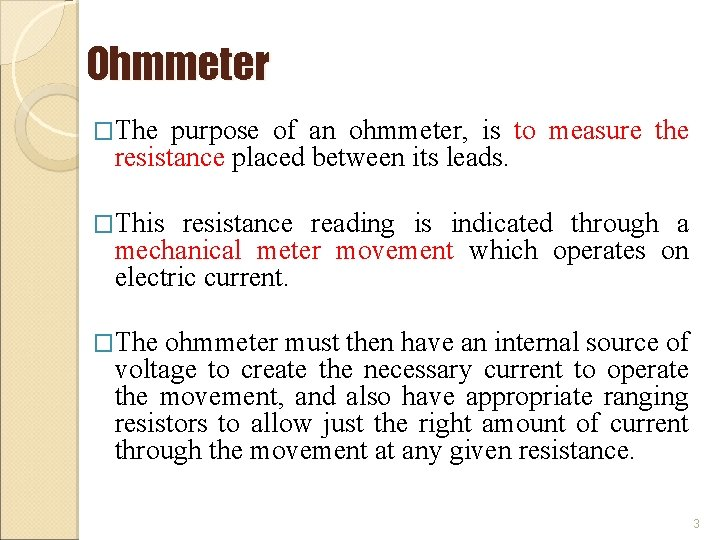Ohmmeter �The purpose of an ohmmeter, is to measure the resistance placed between its leads. �This resistance reading is indicated through a mechanical meter movement which operates on electric current. �The ohmmeter must then have an internal source of voltage to create the necessary current to operate the movement, and also have appropriate ranging resistors to allow just the right amount of current through the movement at any given resistance. 3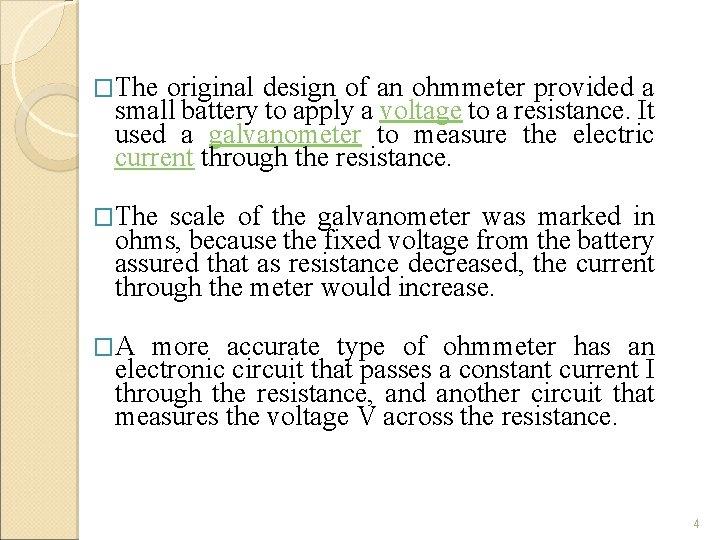�The original design of an ohmmeter provided a small battery to apply a voltage to a resistance. It used a galvanometer to measure the electric current through the resistance. �The scale of the galvanometer was marked in ohms, because the fixed voltage from the battery assured that as resistance decreased, the current through the meter would increase. �A more accurate type of ohmmeter has an electronic circuit that passes a constant current I through the resistance, and another circuit that measures the voltage V across the resistance. 4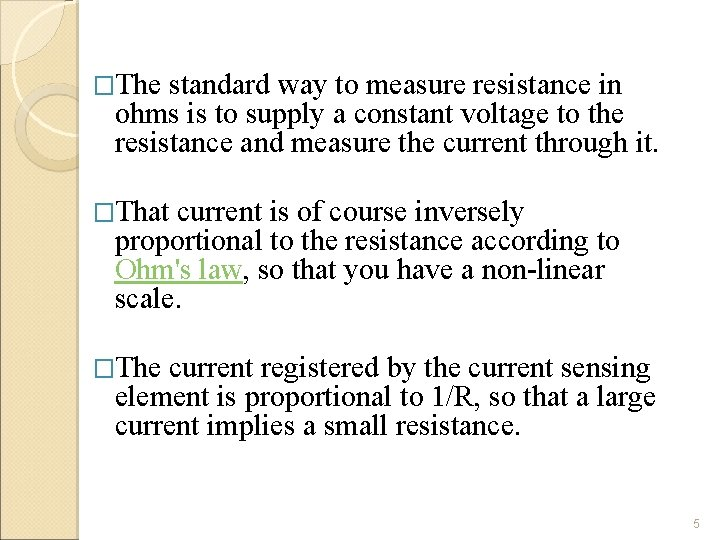�The standard way to measure resistance in ohms is to supply a constant voltage to the resistance and measure the current through it. �That current is of course inversely proportional to the resistance according to Ohm's law, so that you have a non-linear scale. �The current registered by the current sensing element is proportional to 1/R, so that a large current implies a small resistance. 5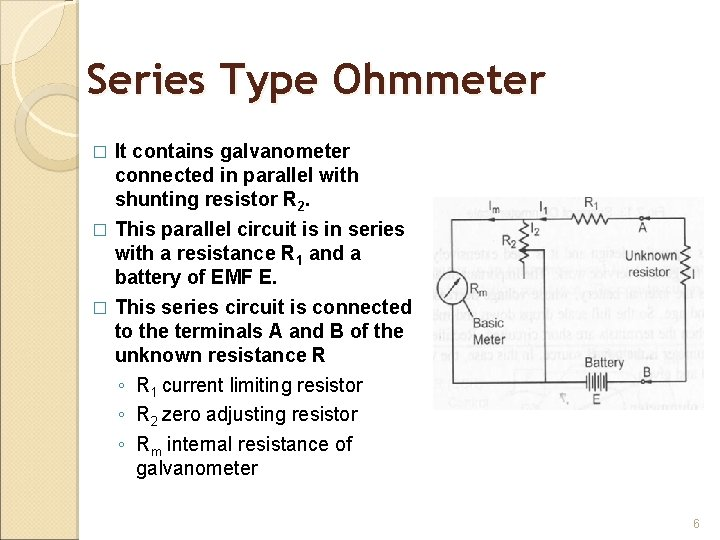Series Type Ohmmeter It contains galvanometer connected in parallel with shunting resistor R 2. � This parallel circuit is in series with a resistance R 1 and a battery of EMF E. � This series circuit is connected to the terminals A and B of the unknown resistance R ◦ R 1 current limiting resistor ◦ R 2 zero adjusting resistor ◦ Rm internal resistance of galvanometer � 6Operation � Supply is given through the battery B and voltage E is supplied. � If terminal A and B are short circuited by shunting the resistance R, maximum power flows through the meter. Under this condition, resistor R 2 is adjusted until the pointer indicates full scale current Ifs. The full scale current position of the pointer is marked ‘ 0’ Ω on the scale. � When R is removed from the circuit, the terminals are open, the movement indicates zero, which is marked infinite ‘ ’ for zero current. � Intermediate scale markings may be placed on the scale by different known values of resistance ‘R’ in the instrument. The accuracy of these scale markings depends on the movement and the tolerance of the calibrating resistors. 7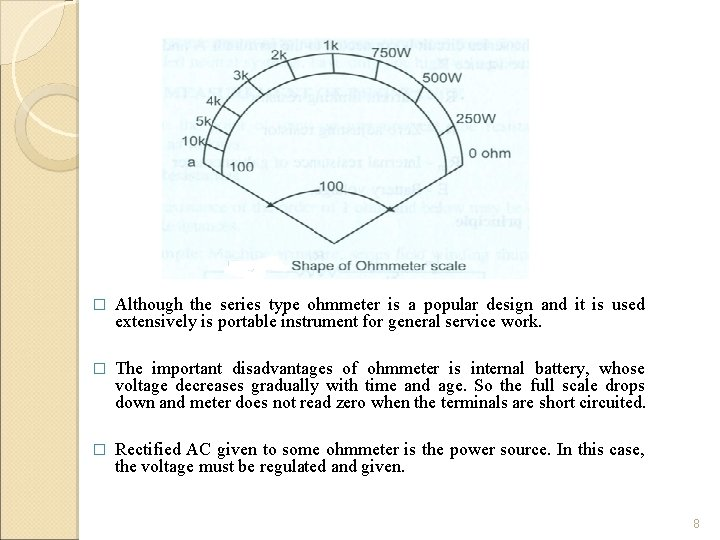� Although the series type ohmmeter is a popular design and it is used extensively is portable instrument for general service work. � The important disadvantages of ohmmeter is internal battery, whose voltage decreases gradually with time and age. So the full scale drops down and meter does not read zero when the terminals are short circuited. � Rectified AC given to some ohmmeter is the power source. In this case, the voltage must be regulated and given. 8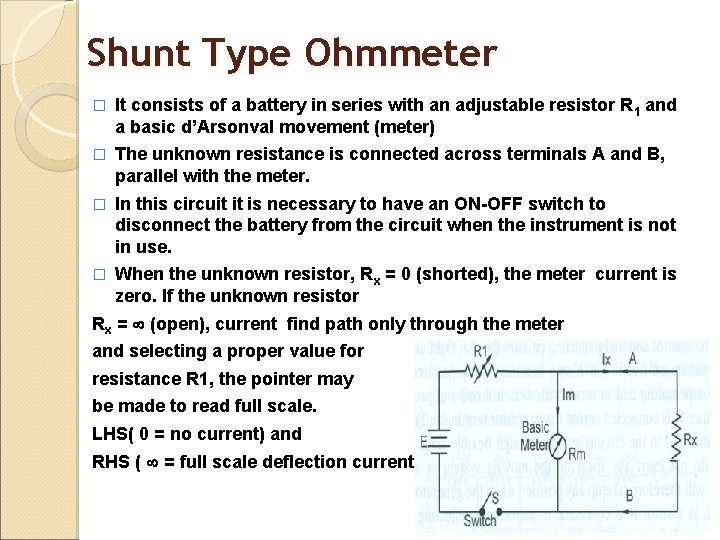Shunt Type Ohmmeter � It consists of a battery in series with an adjustable resistor R 1 and a basic d’Arsonval movement (meter) � The unknown resistance is connected across terminals A and B, parallel with the meter. � In this circuit it is necessary to have an ON-OFF switch to disconnect the battery from the circuit when the instrument is not in use. � When the unknown resistor, Rx = 0 (shorted), the meter current is zero. If the unknown resistor Rx = (open), current find path only through the meter and selecting a proper value for resistance R 1, the pointer may be made to read full scale. LHS( 0 = no current) and RHS ( = full scale deflection current ) 9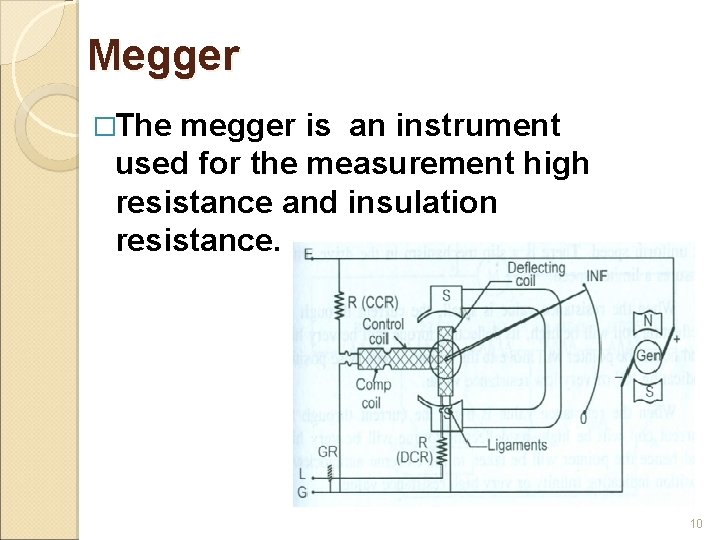Megger �The megger is an instrument used for the measurement high resistance and insulation resistance. 10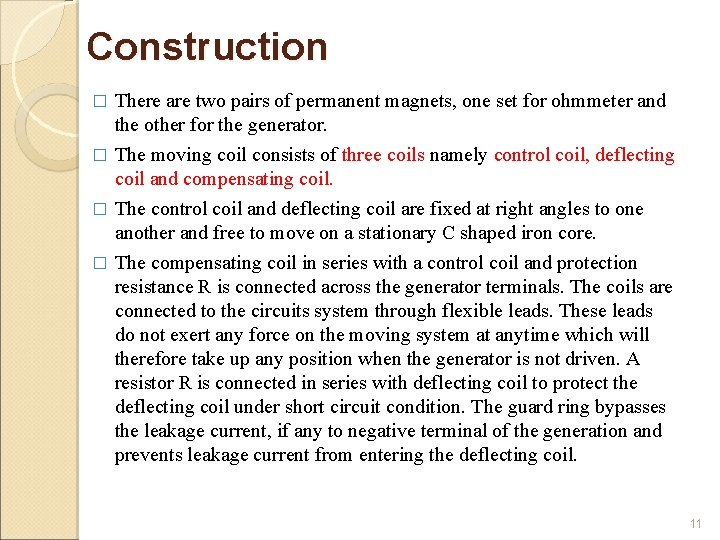Construction There are two pairs of permanent magnets, one set for ohmmeter and the other for the generator. � The moving coil consists of three coils namely control coil, deflecting coil and compensating coil. � The control coil and deflecting coil are fixed at right angles to one another and free to move on a stationary C shaped iron core. � The compensating coil in series with a control coil and protection resistance R is connected across the generator terminals. The coils are connected to the circuits system through flexible leads. These leads do not exert any force on the moving system at anytime which will therefore take up any position when the generator is not driven. A resistor R is connected in series with deflecting coil to protect the deflecting coil under short circuit condition. The guard ring bypasses the leakage current, if any to negative terminal of the generation and prevents leakage current from entering the deflecting coil. � 11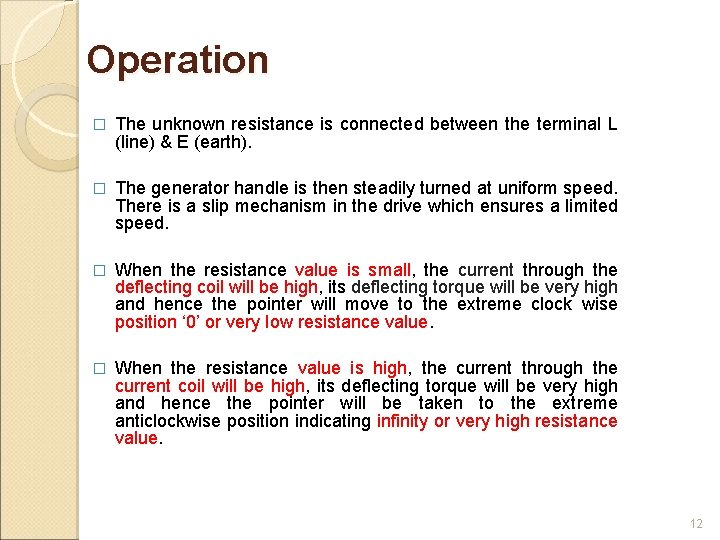Operation � The unknown resistance is connected between the terminal L (line) & E (earth). � The generator handle is then steadily turned at uniform speed. There is a slip mechanism in the drive which ensures a limited speed. � When the resistance value is small, the current through the deflecting coil will be high, its deflecting torque will be very high and hence the pointer will move to the extreme clock wise position ‘ 0’ or very low resistance value. � When the resistance value is high, the current through the current coil will be high, its deflecting torque will be very high and hence the pointer will be taken to the extreme anticlockwise position indicating infinity or very high resistance value. 12Multimeter � A multimeter or a multitester is an electronic measuring instrument that combines several functions in one unit. � Multimeter is basically a PMMC meter. � The most basic instruments include an ammeter, voltmeter, and ohmmeter with a function switch. 13DC Ammeter Section �DC currents are measured making use a suitably designed shunt resistors. 14Multirange Ohm-meter �Multirange ohm-meter is built with the meter movement, battery cells, shunt and series resistors 15DC Voltmeter Section �The meter movement has a resistance of 2000 ohms. �Suitable resistor are added as multiplier to get voltage range from 2. 5 V to 250 V. 16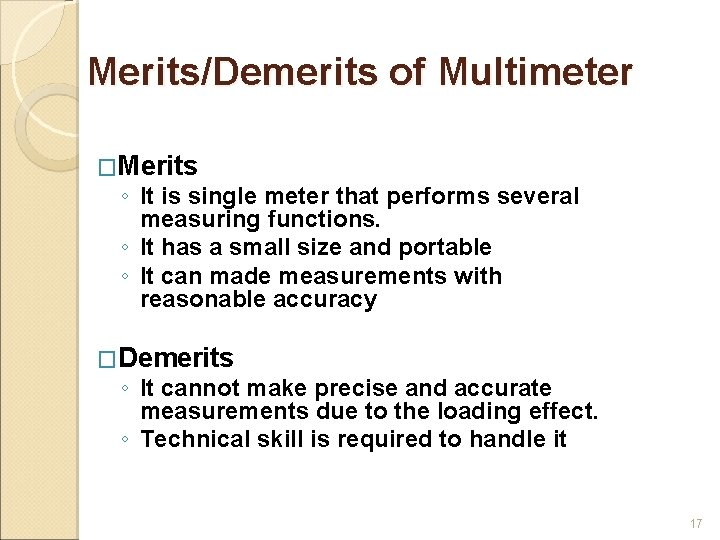Merits/Demerits of Multimeter �Merits ◦ It is single meter that performs several measuring functions. ◦ It has a small size and portable ◦ It can made measurements with reasonable accuracy �Demerits ◦ It cannot make precise and accurate measurements due to the loading effect. ◦ Technical skill is required to handle it 17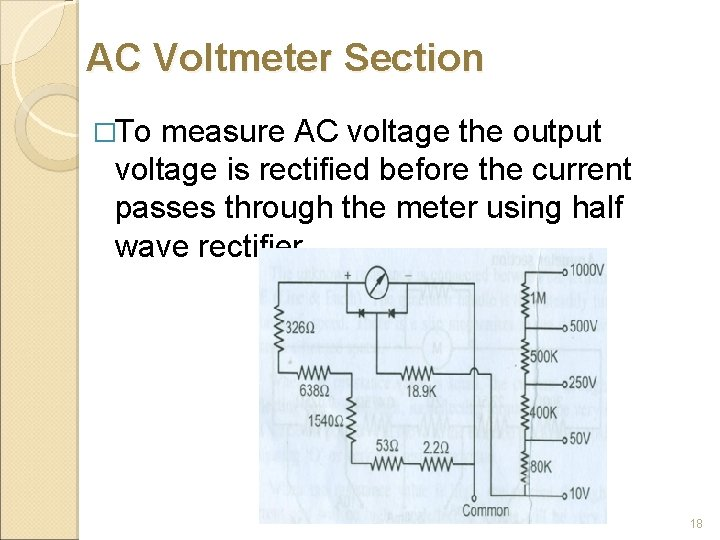AC Voltmeter Section �To measure AC voltage the output voltage is rectified before the current passes through the meter using half wave rectifier. 18Multimeter – Capabilities � � � DC Voltage Measurements � DC and AC Current Measurements � Resistance Measurements � Capacitance/Inductance Measurements � Frequency/Period Measurements � Diode Measurements AC Voltage RMS Measurements 19- End – be continue…Chapter 3 20